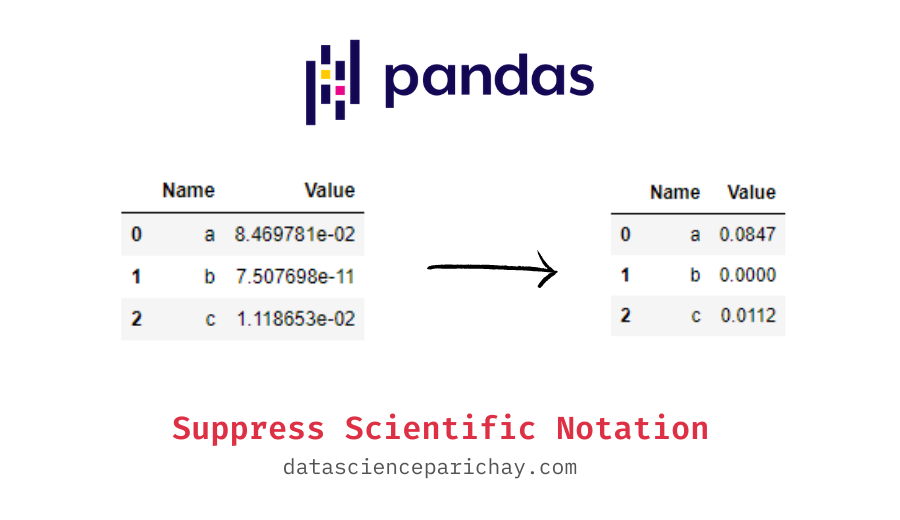# Format Scientific Notation for Floats in Pandas

Sometimes pandas dataframes show floating-point values in scientific notation. This happens particularly when we have values with a high number of decimal points. For example, very small values like 0.000000013. This tutorial will look at how to format scientific notation of floats in a pandas dataframe to their normal notation.

Scientific notation is used to display very large or very small numbers in an easy-to-understand manner. For example, the number 0.000000013 can be represented as `1.3e-08` in scientific notation.

Let’s look at how such values shown in a pandas dataframe by default. For this, we will create a sample dataframe that we will be using throughout this tutorial.

```import numpy as np
import pandas as pd

# create a dataframe
df = pd.DataFrame({
'Name': ['a', 'b', 'c'],
'Value': np.random.rand(3)**20
})
# display the dataframe
df```

Output:

You can see that the floating-point values present in the column “Value” are shown scientific notation. This is because the values are quite small (resulting from an exponent of 20 to random values in 0 to 1).

This may not necessarily be a problem but you may have different formatting preferences. For example, what if you only want the see the real numbers to let’s say 4 decimal places. Let’s look at how to change the formatting of floats appearing in pandas dataframe through some examples.

You can use the pandas `set_option()` function to change the display settings of a pandas dataframe. This function allows you to change a range of options. For this tutorial, we will focus on how to suppress scientific notation of floating-point values appearing in the dataframe.

Let’s go ahead and format the display settings to show only four decimal places in the above dataframe.

📚 Data Science Programs By Skill Level

Introductory

Intermediate ⭐⭐⭐

🔎 Find Data Science Programs 👨‍💻 111,889 already enrolled

Disclaimer: Data Science Parichay is reader supported. When you purchase a course through a link on this site, we may earn a small commission at no additional cost to you. Earned commissions help support this website and its team of writers.

```# show floats to only 4 deicmal places
pd.set_option('display.float_format', lambda x: '%0.4f' % x)
# display the dataframe
df```

Output:

You can see that now the floating-point values are shown with four decimal points and not with scientific notation.

Here we used a lambda function for the float format. You can also use a format string instead to accomplish the same thing.

```# show floats to only 4 deicmal places
pd.set_option('display.float_format', '{:.4f}'.format)
# display the dataframe
df```

Output:

You can see that we get the exact same formatting as with the lambda function.

Note that when using the pandas `set_option()` function only the formatting of the values has changed and not the values themselves. For example, if we individually print one of the values, we see that it is completely intact.

```# first value in the column "Value"
df['Value']```

Output:

`1.3752755941860051e-05`

Also note, that using `pd.set_option()` changes the settings globally. That is, these settings would apply to all the dataframes. You can, however, revert back to the default settings using the pandas `reset_option()` function.

```# reset the display settings back to default
pd.reset_option('display.float_format')
# show the dataframe
df```

Output:

The values are now shown with their default formatting.

For more on the pandas set_option() function, refer to its documentation.

With this, we come to the end of this tutorial. The code examples and results presented in this tutorial have been implemented in a Jupyter Notebook with a python (version 3.8.3) kernel having pandas version 1.0.5

•